### Introduction to Linear Algebra

Throughout this chapter, we’ve seen how linear algebra can be used to tackle systems of linear equations. This is important because of the various contexts in which systems of linear equations appear: even besides areas of math, several areas of physics rely on our ability to solve these systems.

One important area is circuits, which measures how electricity flows throughout various devices. Mathematically analyzing these circuits is extremely important for evaluating their efficacy: too little power into a device and the device won’t work; too much, and the device will overload. As we’ll see throughout this quiz, linear algebra provides the toolbox for analyzing circuits.

# Application: Kirchhoff and Circuits

Let’s first define some of the basic modules present in circuits. In order to get to the actual math part, we won’t go through all of them, but we’ll touch on the important ones. Let’s start our exploration by looking at voltage (rather than current just yet).

First, the basic idea is that current travels through wires, in such a way that the voltage of each contiguous segment of wire (measured in volts, or V) is the same. In other words, there is no change in the potential across any part of the wire. When two or more circuit elements meet, we refer to that point as a node. Since the potential across a given wire segment is assumed constant, we can include the wire joining two or more circuit elements in the definition of a node. For example, the following circuit has two nodes, each with their own voltage:Here, the boxes represent non-wire modules that we’ll look at shortly

How many nodes are in the following circuit?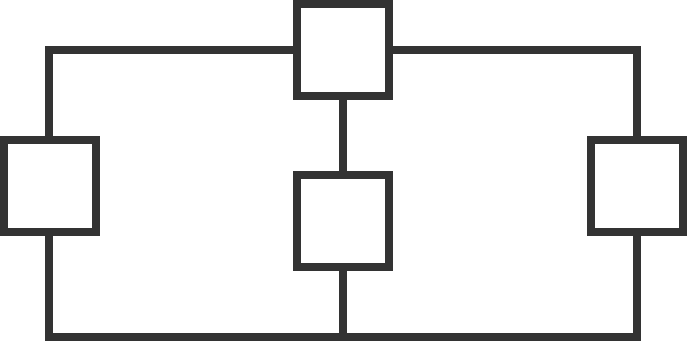# Application: Kirchhoff and Circuits

The main purpose of analyzing circuits is to determine which nodes have which voltages, which in turn tells us how the voltage changes across modules, and thus tells us how much voltage the module uses.

The first of these modules is a simple battery, or voltage source. A battery with voltage $v$ enforces the difference between the positive node (the node connected to the positive part of the battery) and the negative node (the node connected to the negative part of the battery). For example,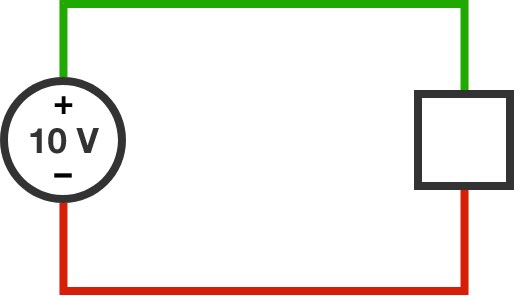Here, the difference between the voltages at the green node and the red node is 10 volts.

Kirchhoff's voltage rule: The sum total of potential changes along a closed circuit is $0.$

The circuit below contains three batteries, with voltages $a, b,$ and $c.$ Use Kirchhoff's voltage rule to determine which of the following must be true.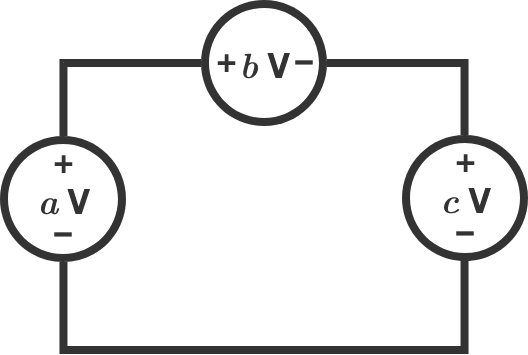Hint: Think of traveling around the circuit once clockwise. When the wire enters a battery from the negative side and exits from the positive side, the total potential goes up by the value of the battery; when a wire enters through the positive side and exits the negative side, the potential goes down by the value of the battery.

# Application: Kirchhoff and Circuits

Before we look at our next module, we have to introduce the idea of current (measured in amperes, or A). Current is the flow of electricity through wires, which can in turn power modules such as lights. As such, current travels over modules as well as through wires.

An important property of current is Kirchhoff’s law: the amount of current going into a node is the same as the amount of current going out of a node. For instance, one possible circuit could be the following: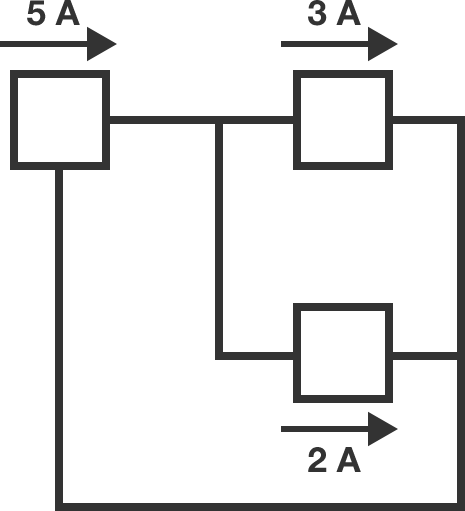# Application: Kirchhoff and Circuits

We are now ready to introduce the second major module we’ll be working with: a resistor. If we apply the same potential difference across geometrically similar conductors, very different currents can result. The resistance of a conductor $($measured in ohms, or $\Omega),$ is given by $\frac{v}{i} = R,$ where $v$ is the applied voltage difference and $i$ is the resulting current. Not all conductors have constant $R ,$ but it is usually a very good approximation in common applications.

An ideal resistor (or Ohmic conductor) is a circuit element obeying Ohm’s law $v = iR,$ where $R$ is constant.

As an example, consider the following circuit, which contains a battery and two resistors of strengths $3 \Omega$ and $2 \Omega,$ respectively.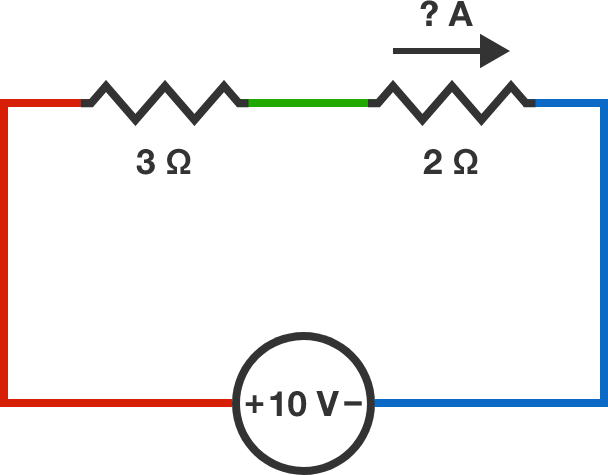Current enters the negative side of the battery and exits the positive side as it makes a clockwise journey around the closed circuit. When it passes through the battery, the charges in the current gain potential, which has to be lost across the resistors according to Kirchhoff's voltage law. $\\\\$

Let's say that the red node (left) is at voltage $v_{\text{red}},$ the green node (middle) is at voltage $v_{\text{green}},$ and the blue node (right) is at voltage $v_{\text{blue}}.$ Then according to Ohm's law, the current across the $3 \Omega$ resistor is $i_{3} = -\frac{v_{\text{green}}-v_{\text{red}}}{3}=\frac{v_{\text{red}}-v_{\text{green}}}{3}.$

According to Kirchhoff's current law, the current traveling across the $3 \Omega$ resistor is the same as the current flowing across the $2 \Omega$ resistor. What is the value of this current?

# Application: Kirchhoff and Circuits

The last few problems illustrate a remarkable fact: using just batteries and resistors, we can induce a current across a module, which allows the module to use that current for power. This is why circuits are so important!

Unfortunately, circuits are usually much more complicated than the simple examples we’ve seen here. In the next couple problems, we’ll work through a much more complex circuit--and see how linear algebra ties into the analysis.

# Application: Kirchhoff and Circuits

Over the next few problems, we’ll analyze the following complex circuit: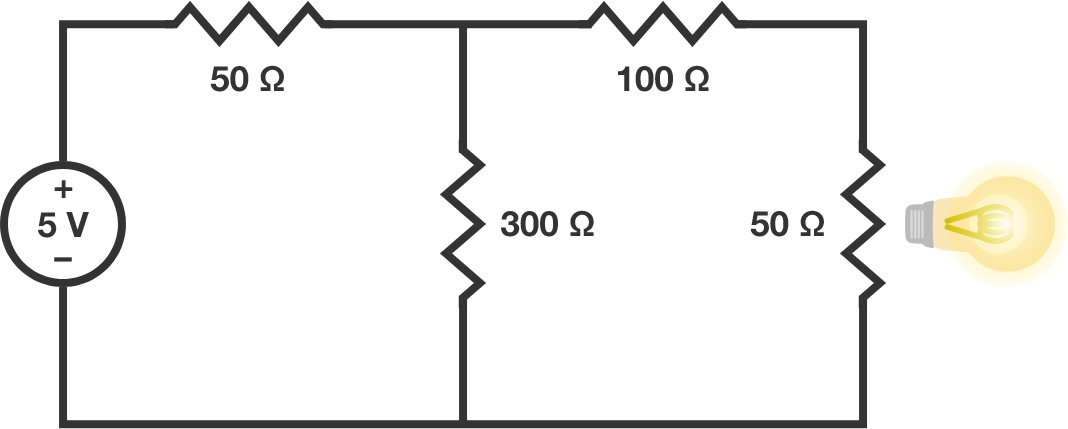Here, a lightbulb is modeled as a $50 \Omega$ resistor. Our goal will be to find the current travelling across the bulb (remember, if the current is too little, the bulb won’t turn on; if the current is too much, the bulb will burn out).

First of all, how many nodes are in this circuit?

# Application: Kirchhoff and Circuits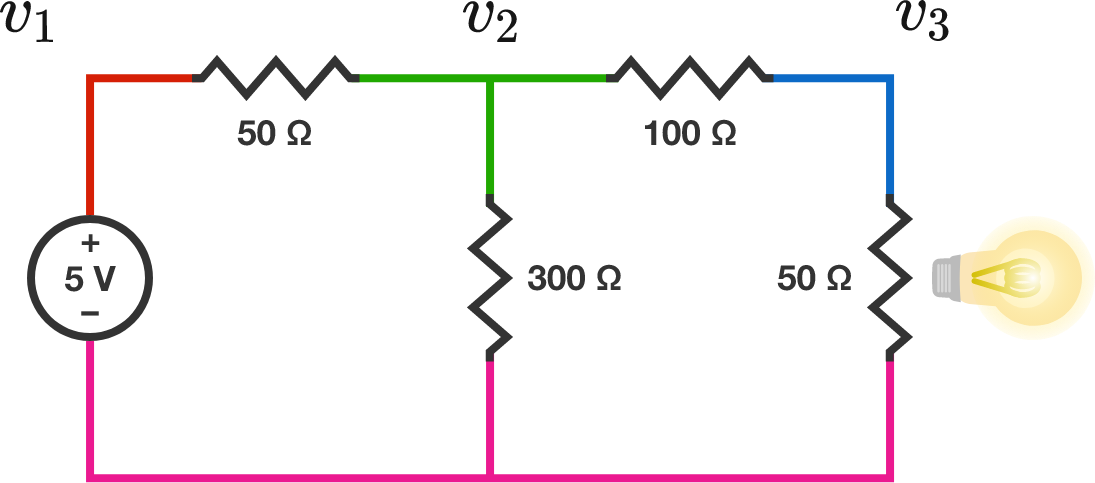From the previous problem, we see there are $4$ nodes. We can assume for simplicity that the pink node has $0$ V voltage. This means there are three nodes we don’t know the voltage of, and thus there are $3$ variables we will need to solve for. As we’ve seen previously, this means we need to find $3$ equations linking them.

Suppose the red node has voltage $v_1$ V, the green node has voltage $v_2$ V, and the blue node has voltage $v_3$ V. Analyzing the red node first, which of the following is true?

# Application: Kirchhoff and CircuitsNow let’s look at a more complicated situation, namely the green node.

Recall that Ohm’s law allows us to write the currents in terms of $v_1, v_2$, and $v_3$. For instance, the current across the $50 \Omega$ resistor flowing from the red node to the green node is $\frac{v_2 - v_1}{50};$ i.e. the potential difference between the green and red nodes divided by the resistance.

There are three currents to worry about if we focus on the green node. Find the three currents and apply Kirchoff’s law to the green node. Which of the following expressions must equal $0?$

Hint: Negative currents flowing out is the same thing as positive currents flowing in.

# Application: Kirchhoff and CircuitsIn the last problem, we saw that applying Ohm’s and Kirchhoff’s laws to the green node gives $\frac{v_2 - v_1}{50} + \frac{v_2 - 0}{300} + \frac{v_2 - v_3}{100} = 0,$ which $($after multiplying both sides of the equal sign by $300$ and simplifying$)$ can be rewritten as $-6v_1 + 10v_2 - 3v_3 = 0.$ Applying the same process to the blue node, which of the following equations is true?

# Application: Kirchhoff and CircuitsWe’ve now reduced to a system of three equations: $\begin{pmatrix}1&0&0\\-6&10&-3\\0&-1&3\end{pmatrix}\begin{pmatrix}v_1\\v_2\\v_3\end{pmatrix} = \begin{pmatrix}5\\0\\0\end{pmatrix}.$ Now we can finally find the current traveling across the bulb. What is this current?

# Application: Kirchhoff and Circuits

In this chapter, we explored the mathematical analysis of circuits, which is integral to correctly setting up electrical systems. As we saw, using just the simple modules of batteries and resistors, we are able to induce a current that can power a lightbulb (or many other things, like the computer you are reading this on!).

As we saw, simple circuits can be analyzed “by hand,” but more complex circuits require a chunk of linear algebra to fully understand. Fortunately, the tools we’ve developed throughout this chapter reduce the problem to a systematic process, from which we can understand precisely what setup is necessary to, for example, light a bulb.

# Application: Kirchhoff and Circuits

×